×

Search anything:

# Types of Generative Adversarial Networks (GANs)

#### Machine Learning (ML) Deep LearningGet this book -> Problems on Array: For Interviews and Competitive Programming

In this article, we cover the types of GAN's. A Generative Adversarial Network is a machine learning algorithm that is capable of generating new training datasets. To explain it briefly , the GANs are made up of two internal submodels namely the generator and the discriminator. The generator creates fake samples using random noise and the discriminator on the other hand diffrentiates between the fake and real samples, after multiple sample are diffrentiated the generator also refers the feedbacks given from discriminator and enhances the fake sample such that the real and fake sample both can't be diffrentiated easily. To read more about the GANs you could refer this article by Taru Jain from opengenus Beginner's Guide to Generative Adversarial Networks with a demo.

There are multiple types of GANs that perform different applications but in this article we are only going to discuss some of the important GANs.

The Different Types of Generative Adversarial Networks (GANs) are:

• Vanilla GAN
• Conditional Gan (CGAN)
• Deep Convolutional GAN (DCGAN)
• CycleGAN
• Generative Adversarial Text to Image Synthesis
• Style GAN
• Super Resolution GAN (SRGAN)

Let us explore details on each type.

1. Vanilla GAN - The Vanilla GAN is the simplest type of GAN made up of the generator and discriminator , where the classification and generation of images is done by the generator and discriminator internally with the use of multi layer perceptrons. The generator captures the data distribution meanwhile , the discriminator tries to find the probability of the input belonging to a certain class, finally the feedback is sent to both the generator and discriminator after calculating the loss function , and hence the effort to minimize the loss comes into picture.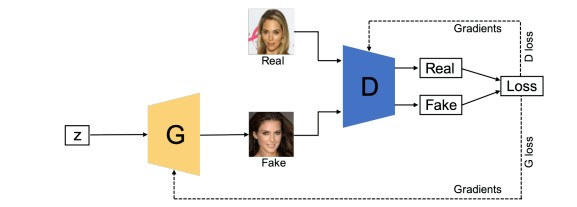2. Conditional Gan (CGAN) - In this GAN the generator and discriminator both are provided with additional information that could be a class label or any modal data. As the name suggests the additional information helps the discriminator in finding the conditional probability instead of the joint probability.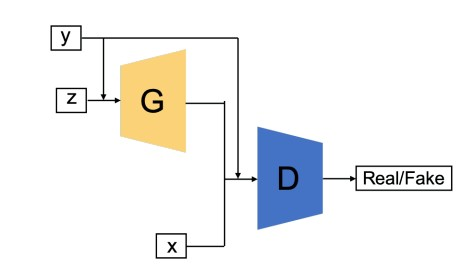The loss function of the conditional GAN is as below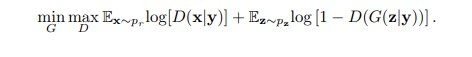3. Deep Convolutional GAN (DCGAN)-This is the first GAN where the generator used deep convolutional network , hence generating high resolution and quality images to be diffrentiated.ReLU activation is used in Generator all layers except last one where Tanh activation is used, meanwhile in Discriminator all layers use the Leaky-ReLu activation function. Adam optimizer is used with a learning rate of 0.0002.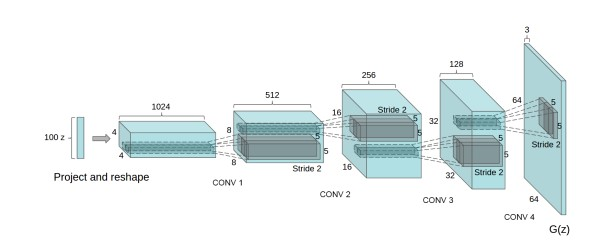The above figure shows the architecture of generator of the GAN. The input generated is of 64 X 64 resolution.

4. CycleGAN - This GAN is made for Image-to-Image translations, meaning one image to be mapped with another image. For example , if summer and winter are made to undergo the process of Image-Image translation we find a mapping function that could convert summer images into that of winter images and vice versa by adding or removing features according to the mapping function,such that the predicted output and actual output have minimized loss.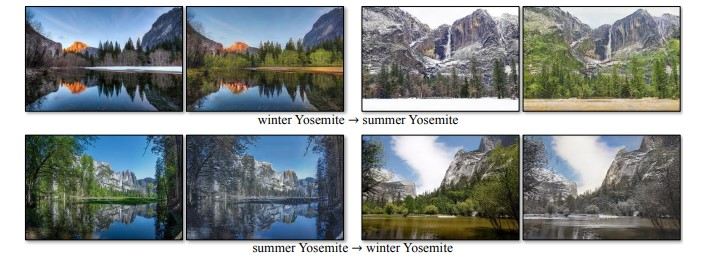5. Generative Adversarial Text to Image Synthesis - In this the GANs are capable of finding an image from the dataset that is closest to the text description and generate similar images.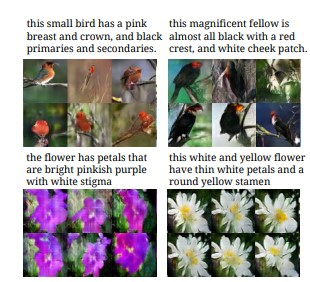The GAN architecture in this case is given below: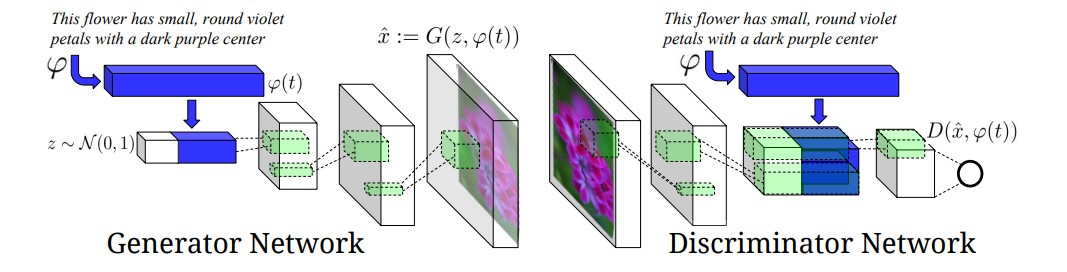As you can the generator network is trying to generate based on the description and the diffrentiation is done by the discriminator based off the features mentioned in text description.

6. Style GAN- Other GANs focused on improving the discriminator in this case we improve the generator. This GAN generates by taking a reference picture.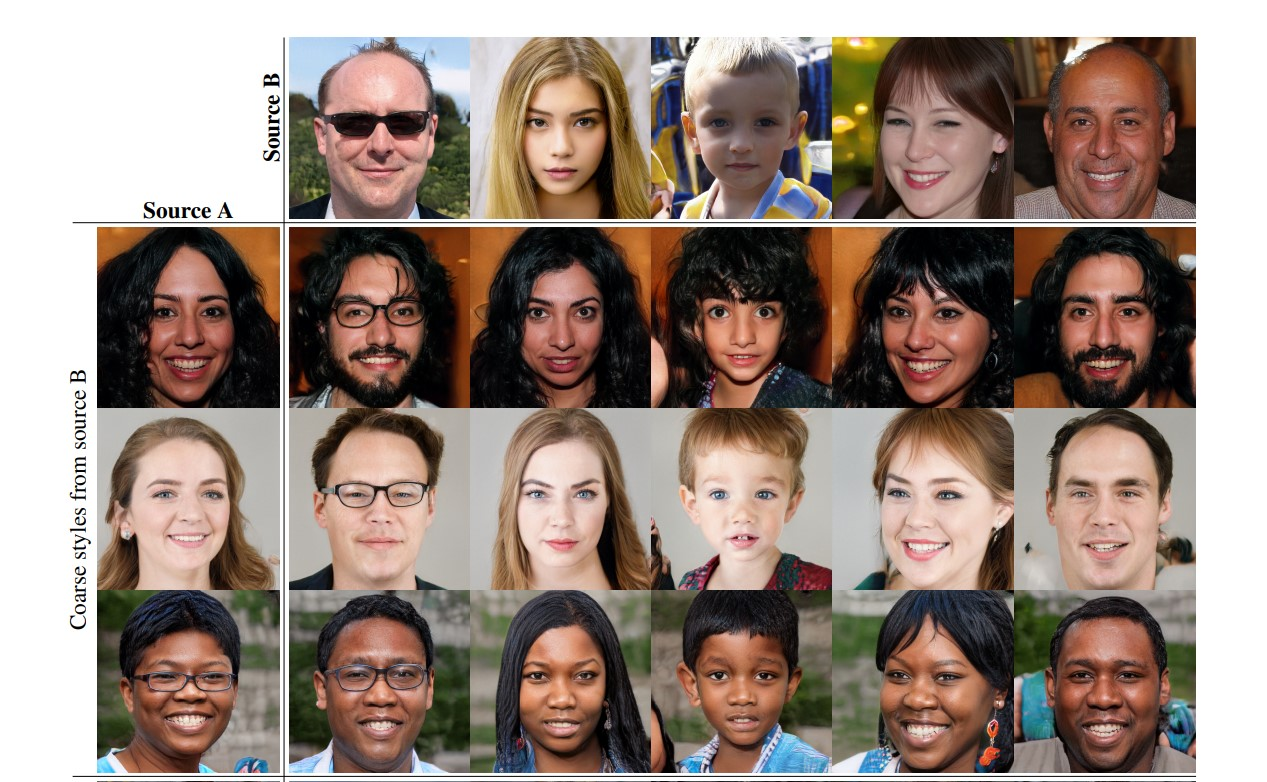As you an see the figure below, Style Gan architecture consists of a Mapping network that maps the input to an intermediate Latent space, Further the intermediate is processed using the AdaIN after each layer , there are approximately 18 convolutional layers.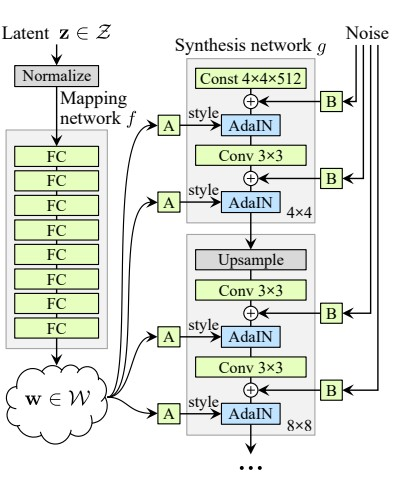The Style GAN uses the AdaIN or the Adaptive Instance Normalization which is defined as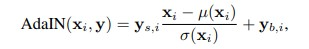Here, xi is the feature map , which is tweaked by using the component y.

7. Super Resolution GAN (SRGAN)- The main purpose of this type of GAN is to make a low resolution picture into a more detailed picture. This is one of the most researched problems in Computer vision. The architecture of the SRGAN is given below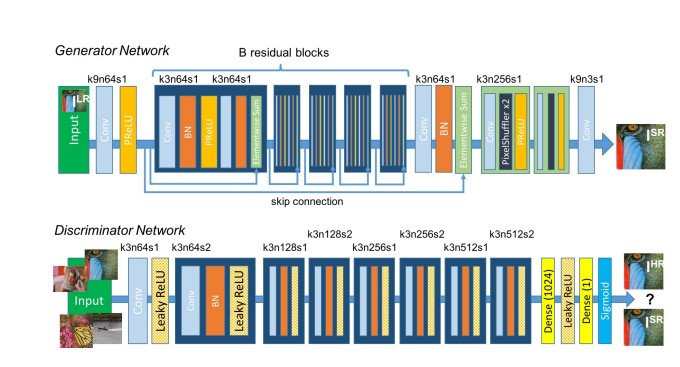As given in the above figure we observe that the Generaor network and Discriminator both make use of Convolutional layers , the Generator make use of the Parametric ReLU as the activation function whereas the Discriminator uses the Leaky ReLU.
The Generator takes the input data and finds the average of all possible solutions that is pixelwise, when formulated looks like,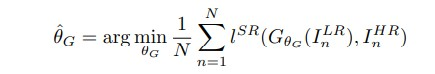here, Ihr represents high resolution image , whereas Ilr represents lowresolution image and lsr represents loss and θg represents the weight and biases at layer L.
Now the perceptual loss is given as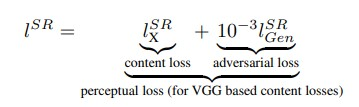Finally the content loss is summed with adversial loss and Mean Squared Error is taken to find the best pixel wise solution.

Applications:

1. Firstly, GANs can be used as data augmentation techniques where the generator generates new images taking the training dataset and producing multiple images by applying some changes.
2. GANs improve the classification techniques by training the discriminator on a very large scale of data that are real as well as fake.
3. GANs are also used to improve the resolution of any input image.
4. Just like filters used in Snapchat , a filter could be applied to see what a place might look like if in summer, winter,spring or autumn and many more conditions could be applied and hence thats where Deep ConvNets play their role.
5. GANs are used to convert semantics into images and better understand the visualizations done by the machine.

FAQs:

1. How does the generator generate images?
Solution : The generator takes noise from latent space and further based on test and trial feedback , the generator keeps improvising on images. After a certain times of trial and error , the generator starts producing accurate images of a certain class which are quite difficult to diffrentiate from real images.

2. How does the discriminator perform classification?
Solution: The discriminator gets a probability score after convolutions and hence the discriminator chooses the decision based on the probability.

#### Anubhav Tewari

Anubhav Tewari has been a Machine Learning Developer, Intern at OpenGenus.

Types of Generative Adversarial Networks (GANs)